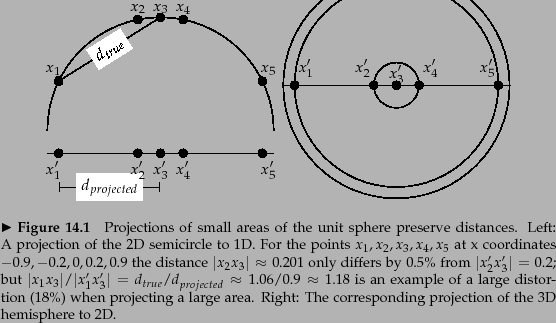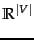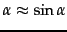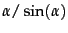Next: Rocchio classification Up: Vector space classification Previous: Vector space classification   Contents   Index

# Document representations and measures of relatedness in vector spacesAs in Chapter 6 , we represent documents as vectors inin this chapter. To illustrate properties of document vectors in vector classification, we will render these vectors as points in a plane as in the example in Figure 14.1 . In reality, document vectors are length-normalized unit vectors that point to the surface of a hypersphere. We can view the 2D planes in our figures as projections onto a plane of the surface of a (hyper-)sphere as shown in Figure 14.2 . Distances on the surface of the sphere and on the projection plane are approximately the same as long as we restrict ourselves to small areas of the surface and choose an appropriate projection (Exercise 14.1 ).

Decisions of many vector space classifiers are based on a notion of distance, e.g., when computing the nearest neighbors in kNN classification. We will use Euclidean distance in this chapter as the underlying distance measure. We observed earlier (Exercise 6.4.4 , page) that there is a direct correspondence between cosine similarity and Euclidean distance for length-normalized vectors. In vector space classification, it rarely matters whether the relatedness of two documents is expressed in terms of similarity or distance.

However, in addition to documents, centroids or averages of vectors also play an important role in vector space classification. Centroids are not length-normalized. For unnormalized vectors, dot product, cosine similarity and Euclidean distance all have different behavior in general (Exercise 14.8 ). We will be mostly concerned with small local regions when computing the similarity between a document and a centroid, and the smaller the region the more similar the behavior of the three measures is.

Exercises.

• For small areas, distances on the surface of the hypersphere are approximated well by distances on its projection (Figure 14.2 ) becausefor small angles. For what size angle is the distortion(i) 1.01, (ii) 1.05 and (iii) 1.1?Next: Rocchio classification Up: Vector space classification Previous: Vector space classification   Contents   Index